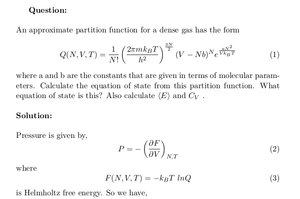Solved Problems in Physics, Physical Chemistry, and Computer Simulation Projects for College and Graduate Students.# Equation of State of a Dense Gas

Regular price
\$1.00
Sale price
\$1.00
Tax included.

Question:

An approximate partition function for a dense gas has the formwhere a and b are the constants that are given in terms of molecular parameters. Calculate the equation of state from this partition function. What equation of state is this? Also calculate <E> and CV .

By purchasing this product, you will get the step by step solution of the above problem in pdf format and the corresponding latex file where you can edit the solution. I am also available to help  you with any possible question you may have.